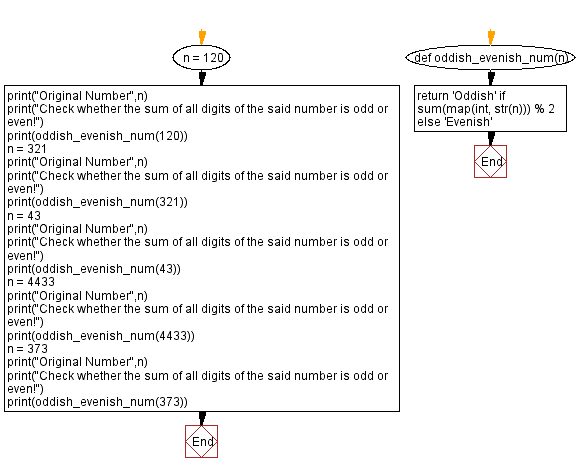﻿ Python: Check whether a given number is Oddish or Evenish - w3resource# Python: Check whether a given number is Oddish or Evenish

## Python Basic - 1: Exercise-107 with Solution

Write a Python program to check whether a given number is Oddish or Evenish.

A number is called "Oddish" if the sum of all of its digits is odd, and a number is called "Evenish" if the sum of all of its digits is even.

Sample Solution:

Python Code:

``````def oddish_evenish_num(n):
return 'Oddish' if sum(map(int, str(n))) % 2 else 'Evenish'
n = 120
print("Original Number",n)
print("Check whether the sum of all digits of the said number is odd or even!")
print(oddish_evenish_num(120))
n = 321
print("Original Number",n)
print("Check whether the sum of all digits of the said number is odd or even!")
print(oddish_evenish_num(321))
n = 43
print("Original Number",n)
print("Check whether the sum of all digits of the said number is odd or even!")
print(oddish_evenish_num(43))
n = 4433
print("Original Number",n)
print("Check whether the sum of all digits of the said number is odd or even!")
print(oddish_evenish_num(4433))
n = 373
print("Original Number",n)
print("Check whether the sum of all digits of the said number is odd or even!")
print(oddish_evenish_num(373))
``````

Sample Output:

```Original Number 120
Check whether the sum of all digits of the said number is odd or even!
Oddish
Original Number 321
Check whether the sum of all digits of the said number is odd or even!
Evenish
Original Number 43
Check whether the sum of all digits of the said number is odd or even!
Oddish
Original Number 4433
Check whether the sum of all digits of the said number is odd or even!
Evenish
Original Number 373
Check whether the sum of all digits of the said number is odd or even!
Oddish
```

Pictorial Presentation:Flowchart:Python Code Editor:

Have another way to solve this solution? Contribute your code (and comments) through Disqus.

What is the difficulty level of this exercise?

Test your Programming skills with w3resource's quiz.

﻿

## Python: Tips of the Day

Iterating over dictionaries using 'for' loops:

I am a bit puzzled by the following code: d = {'x': 1, 'y': 2, 'z': 3} for key in d: print key, 'corresponds to', d[key] What I don't understand is the key portion. How does Python recognize ...

key is just a variable name.

```for key in d:
```

For Python 3.x:

```for key, value in d.items():
```

For Python 2.x:

```for key, value in d.iteritems():
```

To test for yourself, change the word key to poop.

In Python 3.x, iteritems() was replaced with simply items(), which returns a set-like view backed by the dict, like iteritems() but even better. This is also available in 2.7 as viewitems().

The operation items() will work for both 2 and 3, but in 2 it will return a list of the dictionary's (key, value) pairs, which will not reflect changes to the dict that happen after the items() call. If you want the 2.x behavior in 3.x, you can call list(d.items()).

Ref: https://bit.ly/37dm0Qo